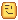# Math.random() how to have decibels?

Hi, while using `math.random()` I thought it would be a good idea since the 2 numbers don’t have a long “distance” between each other.
Here is the code: (just to show the numbers)

``````local random = math.random(1.0,3.0)
``````
1 Like

`local random = math.random(1, 30) / 100`

You can’t have decimals in math.random, to get around this just divide it by 100

10 Likes

It worked. Thanks a lot.2 Likes

math.random certainly allows decimals. When called with no arguments it returns an evenly distributed number between 0 and 1.

The general method to get a random float between numbers A and B is this:

``````math.random() * (B - A) + A
``````

Alternatively you can use the more recently added Random object. It contains functions for generating floats or integers in specified bounds.

2 Likes

roblox has a Random object that provides a much more user friendly interface for math.random

``````local optionalSeed = player.UserId

local r = Random.new(optionalSeed)

for i = 1, 10 do
print(r:NextNumber(1, 100))
warn(r:NextInteger(1, 100))
end
``````

My favorite part is how you construct it with a seed. math.randomseed being global can be such a mess.

1 Like

Worth noting that most of the time the Random object is a better choice than math.random. Because nothing has touched its pseudorandom numbers you will see more consistently random results AFAIK.

EDIT: Just saw you already mentioned math being global. My fault I was just skimming the post.It’s just an interface to the same underlying random from math.c. When they added the Random object, they replaced the pseudorandom algorithm used in math.c cuz the old one was notorious for being awful. It’s XSH-RR now. A Random Feature

1 Like

oh you meant for seeds. Constructing it with a nil seed uses hardware entropy so it’s the most random you can realistically get on a pc. I just like being able to construct separate seeded randoms because you can use it for situations like deterministically generating a unique space ship for each player by using their user id as seed.

randomseed can still do this but it can be a major PITA. like if you had lazy generated content you would need to track the number of random numbers retrieved, then call math.randomseed to switch back to the seed. waste electrons iterating thru and burning the already retrieved random numbers until you get back to the point where you left off. anyone else who needs their next random number has to wait for this one to be finished.

1 Like

`math.random` mirrors the `Random` class

1 Like